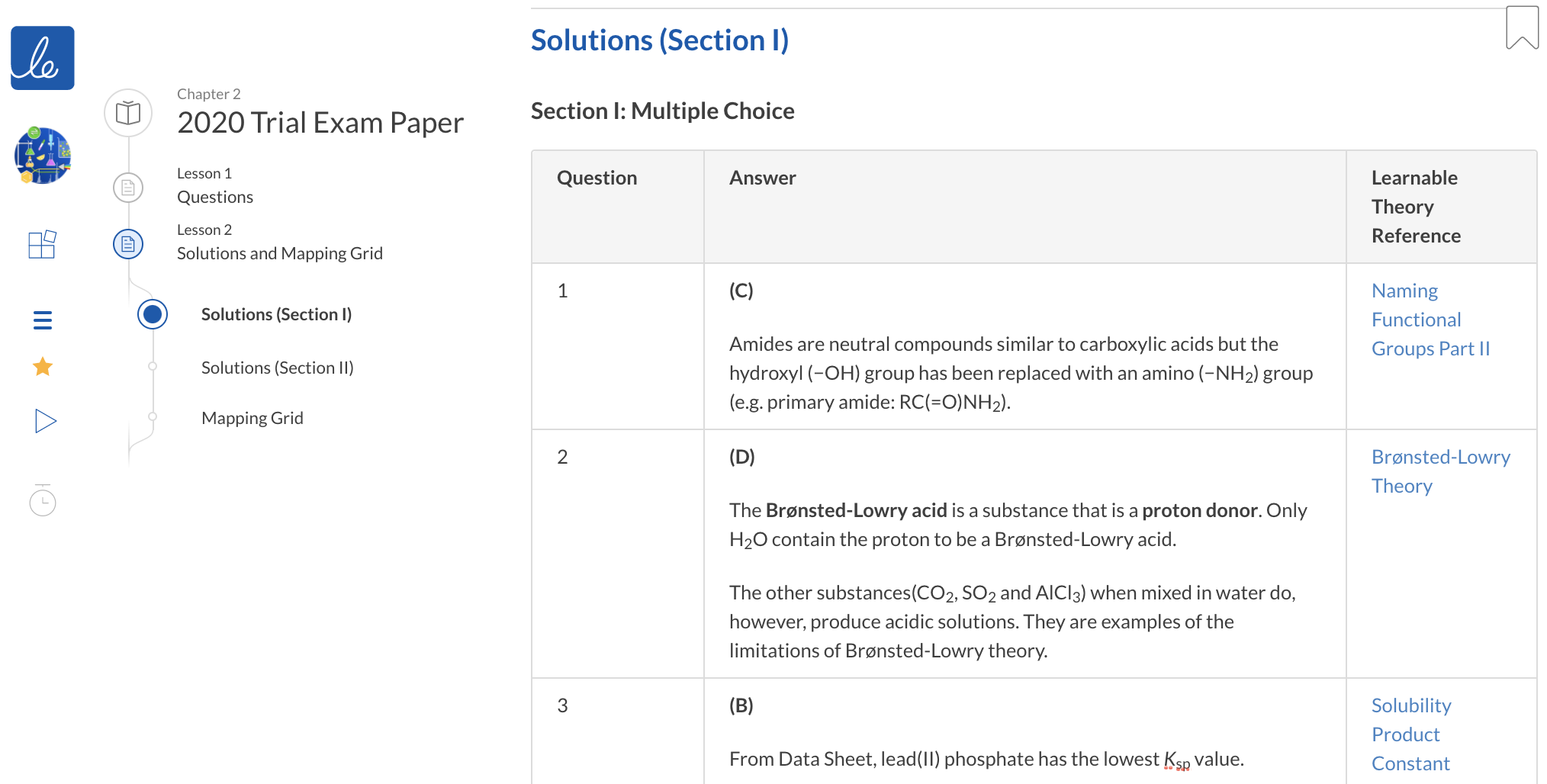# HSC Chemistry Trial Papers | Free 2020 Chemistry Practice Exam

Access a free full HSC Trial Exam Paper with detailed solutions written by experienced HSC Chemistry teachers. This free Chemistry trial paper written for the new syllabus covers modules 5 - 7. We recommend to complete under exam conditions!

## 2020 HSC Chemistry Trial Exam Paper by Learnable

Looking for past trial exam papers for Chemistry ?

This free Chemistry trial paper assesses your understanding of the following modules:

• Module 5: Equilibrium and Acid Reactions
• Module 6: Acid/Base Reactions
• Module 7: Organic Chemistry

This Chemistry trial paper written for the new syllabus covers modules 5 – 7 as most schools do not include Module 8 in their Chemistry trial exam.

## Advice for getting the most out of 2020 HSC Chemistry Trial Exam Paper

It is recommended you complete

1. Module 5 – 7 theory lessons and quizzes on the Year 12 Chemistry course on Learnable before attempting this exam to ensure you perform your best.
2. Read the Exam skills including Common Mistakes & Misconceptions for each module.

The most effective way to finalise your exam preparation for Chemistry is to attempt as many practice papers as possible under exam conditions.

## Instructions for sitting the 2020 HSC Chemistry Trial Exam Paper

1. Working time is 3 hours.
2. Attempt all questions.
3. You must write in black pen. Do not write in pencil.
4. You may only use a pencil for drawing diagrams and graphs.
5. All necessary working should be shown in every question. Marks may be deducted for careless or badly arranged work.
6. Set out all working for numerical questions and provide appropriate units.
7. You may refer to a formulae sheet and data sheet.
8. Calculators approved by NESA may be used.

Copyright © 2020 Learnable Education. All rights reserved. No part of this material may be reproduced, stored in or introduced into a retrieval system or transmitted, in any form, or by any means (electronic, mechanical, photocopying, recording, or otherwise), without the prior permission of Learnable Education.

## Section I: Multiple Choice

In Section I of 2020 Chemistry Trial Paper:

1. There are 20 multiple-choice questions.
2. Each question is worth 1 mark.
3. Allow 35 minutes for this section.

### Question 1

Which structural formula represents pentanamide?

 (A)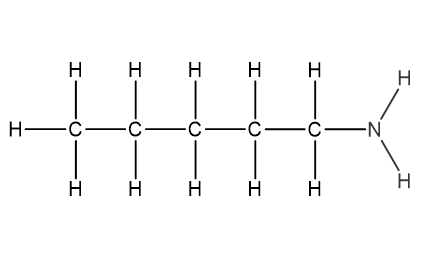(B)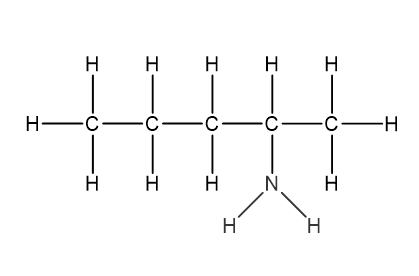(C)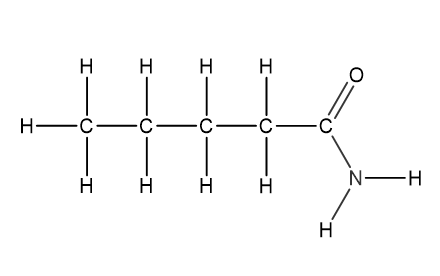(D)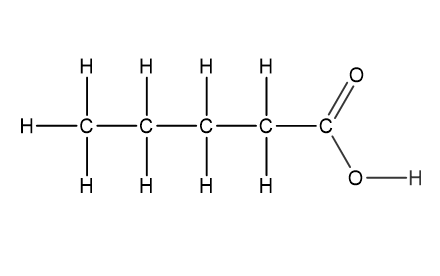### Question 2

Which of the following is a Brønsted-Lowry acid?

(A) CO2

(B) SO2

(C) AlCl3

(D) H2O

### Question 3

Which of the following metal phosphates has the lowest molar solubility?

(A) Barium phosphate

(C) Magnesium phosphate

(D) Calcium phosphate

Use the diagram below to answer Questions 4 and 5.

The diagram represents the conductometric titration curve for an equimolar reaction between an acid (analyte) and a base (titrant).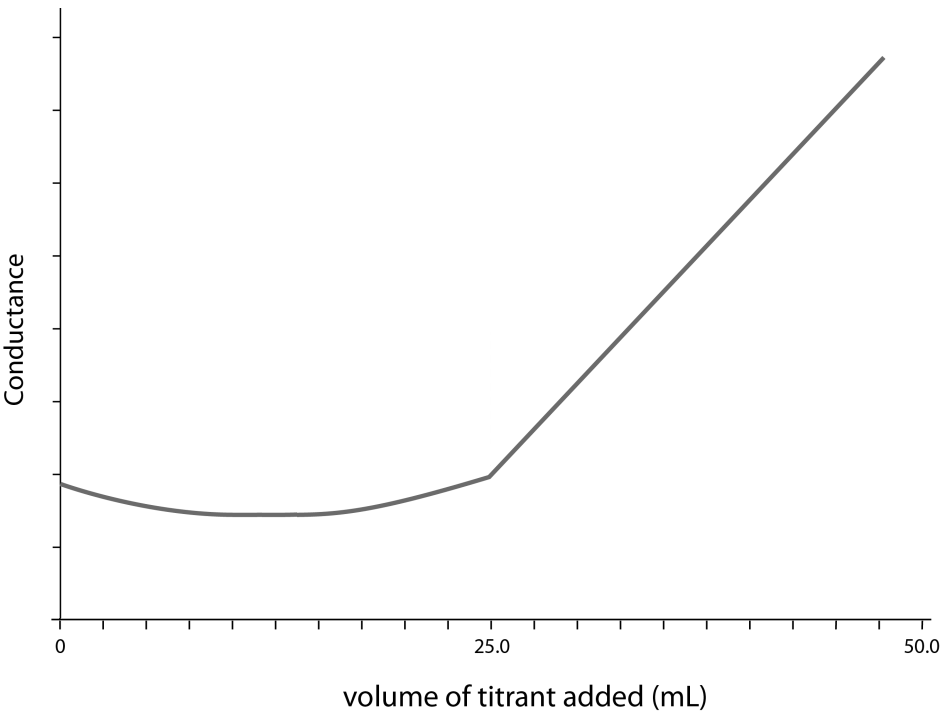### Question 4

Which indicator would be best for this titration?

 Indicator Colour change range (pH) (A) Thymol blue 1.2 − 2.8 (B) Phenol red 6.8 − 8.2 (C) Cresolphthalein 8.2 − 9.8 (D) Indigo carmine 11.6 − 14.0

### Question 5

Which of the following equations best represents the reaction described by the titration curve?

(A) HCl(aq) + KOH(aq) → KCl(aq) + H2O(l)

(B) HCl(aq) + NH3(aq) → NH4Cl(aq)

(C) CH3COOH(aq) +KOH(aq)→ KCH3COO(aq) + H2O(l)

(D) CH3COOH(aq) + NH3(aq) → NH4CH3COO(aq)

### Question 6

All of the following compounds have similar molar mass. Which has the lowest boiling point?

(A) Heptane

(B) Pentanoic acid

(C) Hexan-1-amine

(D) Pentanamide

### Question 7

Hydrogen chloride gas can react with oxygen to form toxic chlorine gas and water vapour.

How does the replacement of pure oxygen with air affect this reversible reaction?

(A) It increases the rate of reaction and increases the yield.

(B) It increases the rate of reaction and decreases the yield.

(C) It decreases the rate of reaction and increases the yield.

(D) It decreases the rate of reaction and decreases the yield.

### Question 8

The structure of an organic compound is shown.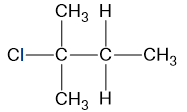Which row of the table correctly gives the name of compound and the reactants used to produce it in a reaction?

 Name Reactant 1 Reactant 2 (A) 2-chloro-2-methylbutane 2-methylbutan-1-ol Hydrogen chloride (B) 2-chloro-2-methylbutane 2-methylbutan-2-ol Hydrogen chloride (C) 1-chloro-1,1-dimethylpropane 1,1-dimethylpropan-1-ol Hydrogen chloride (D) 1-chloro-1,1-dimethylpropane 1,1-dimethylpropan-2-ol Hydrogen chloride

### Question 9

Which of the following compounds have the lowest pKa value?

(A) Methanoic acid

B) Ethanoic acid

(C) Propanoic acid

(D) 2-Methylpropanoic acid

### Question 10

The structure of an organic compound is shown.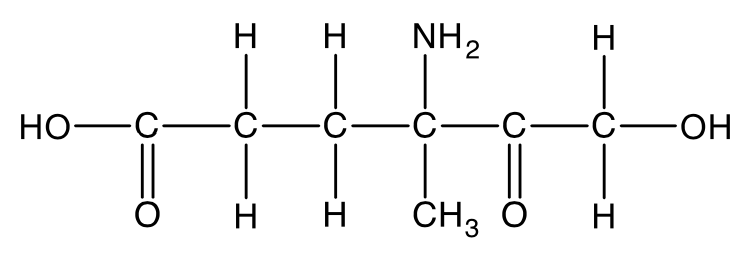Which one of the following functional group is not present in the compound?

(A) Amide

(B) Alcohol

(C) Ketone

(D) Carboxylic acid

### Question 11

Nitrogen dioxide is a toxic brown gas which converts to colourless dinitrogen tetroxide gas in a reversible reaction.

$2NO_{2(g)} \rightleftharpoons N_2O_{4(g)} \kern{1cm} \Delta H < 0$

A mixture of the two gases was sealed within a container.

What would be observed if the the volume of the flask was suddenly decreased?

(A) Equilibrium constant remains the same.

(B) Equilibrium constant increases.

(C) Equilibrium constant decreases.

(D) There is not enough information to determine the change in equilibrium constant.

### Question 12

The concentration profile diagram represents a mixture of three gases, X, Y and Z, in a sealed container at room temperature. At 1.5 minutes, the container was placed in an ice bath.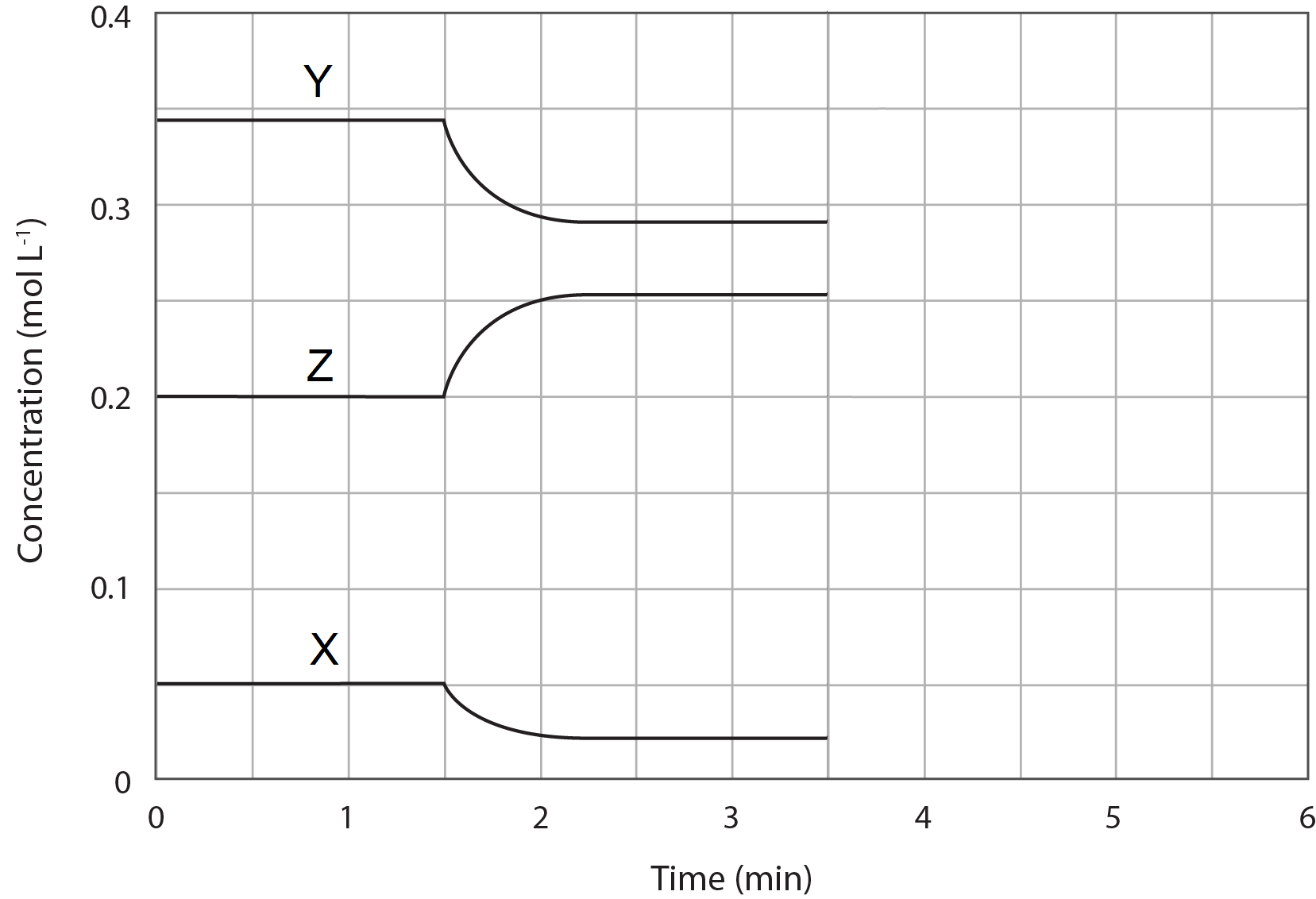Which of the following statement best represents the equilibrium system described by the diagram?

(A) 2Z(g) $\rightleftharpoons$ X(g) + 2Y(g) and the forward reaction is exothermic.

(B) X(g) $\rightleftharpoons$ 2Z(g) + 2Y(g) and the forward reaction is endothermic.

(C) X(g) + 2Y(g) $\rightleftharpoons$  2Z(g)and the forward reaction is exothermic.

(D) ​X(g) + 2Y(g) $\rightleftharpoons$  2Z(g)  and the forward reaction is endothermic.

### Question 13

In an experiment, 15.0 g of bioethanol is produced by the fermentation of glucose.

What is the volume of CO2 gas that would be produced at 25 °C and 100 kPa?

(A) 2.02 L

(B) 4.04 L

(C) 8.07 L

(D) 16.1 L

### Question 14

2-hydroxyethanoic acid forms a polymer with an average molar mass of 5.25 x 104 g mol-1.

Which of the following is the closest number of hydrogen atoms in an average polymer chain?

(A) 700

(B) 900

(C) 1400

(D) 1800

### Question 15

What is the resultant pH of the solution when equal volumes of pH 12.00 potassium hydroxide and pH 12.60 barium hydroxide are mixed?

(A) 12.20

(B) 12.30

(C) 12.40

(D) 12.60

### Question 16

How many different structural isomers have the molecular formula C4H8Br2?

(A) 9

(B) 10

(C) 12

(D) 13

### Question 17

The Contact Process is used industrially to manufacture sulfuric acid. One of the steps in the process involves synthesis of sulfur trioxide which is made reversibly by oxidising sulfur dioxide with oxygen.

400 moles of sulfur dioxide and 600 moles of oxygen gas were placed in 2000 L reactor. At equilibrium, the concentration of sulfur trioxide is 0.095 mol L-1. What is the value of K for the synthesis of sulfur trioxide?

(A) 0.19

(B) 0.75

(C) 3.24

(D) 3.99

### Question 18

What is the solubility in ppm of lead(II) chloride in seawater (19400 ppm of sodium chloride)?

(A) 4.29 x 10-5

(B) 4.29 x 10-2

(C) 14.2

(D) 42.9

### Question 19

When 20 mL of 0.10M KOH is mixed with 10mL of 0.10 M HBr, the temperature of the solution increases by 0.23˚C. Assume that the specific heat capacities of the solutions are equal and constant.

Which of the following best describes the temperature change when 10mL of 0.1M HCl is mixed with 25mL of 0.1M NaOH?

(A) Temperature change is less than 0.23˚C.

(B) Temperature change is greater than 0.23˚C.

(C) Temperature change is equal to 0.23˚C.

(D) There is not enough information to determine the temperature change.

### Question 20

An asymmetric alkene, compound X, with a molecular weight of 56.10 g mol-1 was treated with dilute sulfuric acid and heat to produce two isomeric organic compounds, Y and Z. Treatment of Y with acidified potassium dichromate resulted in colour change of the solution from orange to green, while no colour change was observed when Z was treated with same reagent.

Which of the following correctly identities compound Y?

(A) Butan-2-ol

(B) 2-Methylpropan-1-ol

(C) 2-Methylpropan-2-ol

(D) Butan-2-one

## Section II: Extended Response Questions

In Section II of 2020 Chemistry Trial Paper:

1. There are 15 Extended Response Questions.
2. Allow 2 hours 25 minutes for this section.

### Question 21 (7 marks)

Alkanes, alkenes and alkynes are simple hydrocarbon chains with no functional groups, with alkanes being the simplest organic compounds in organic chemistry.

 (a) Explain the trend in the boiling points of the first five straight-chain alkanes. 2 (b) Define the term homologous series and provide two characteristics of organic compounds in a homologous series. 2 (c) Identify a suitable chemical test to distinguish between alkanes and alkenes and explain the expected observations. 3

### Question 22 (6 marks)

Butyl butanoate is a clear, colourless liquid that has pleasant aroma that is used to add fruity pineapple flavours.

Outline the steps required for the synthesis of butyl butanoate in a school laboratory. Support your answer with a diagram and a chemical equation using structural formulae.

### Question 23 (4 marks)

Many health problems that make our body too acidic or too basic are usually linked to the pH of blood. Our blood contains H2CO3/HCO3 that regulates the pH to not vary by more than 0.2 from the average 7.40.

Hyperventilation is a condition in which you have rapid and deep breathing with more exhaling than inhaling.

Explain the effect of hyperventilation on the pH of blood. Support your answer with at least ONE chemical equations.

### Question 24 (9 marks)

Methanol can be synthesised from carbon monoxide which can be produced from a reverse water-gas shift reaction involving only gaseous components.

The concentration profile diagram of the reaction is given below.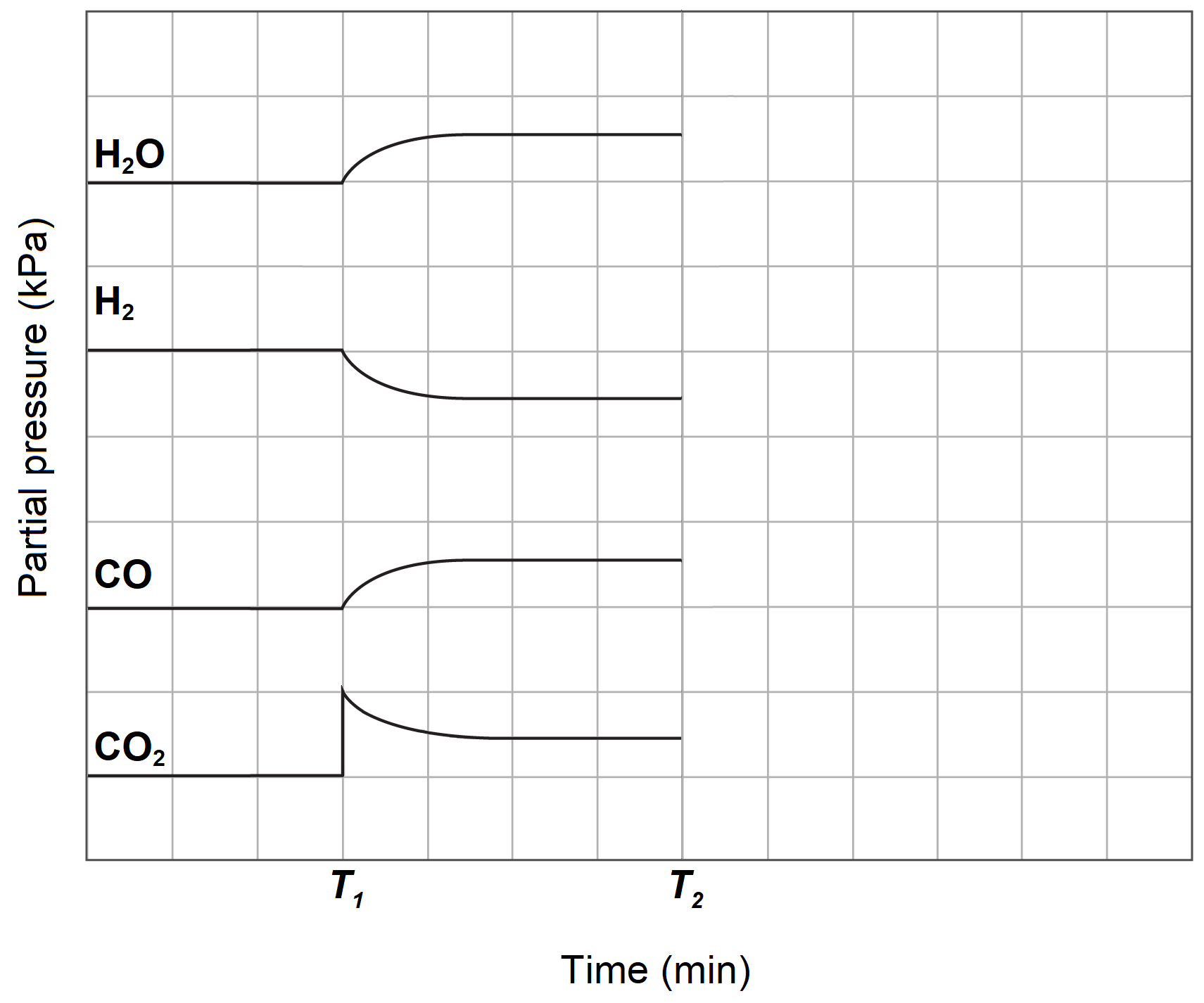At time T1, some carbon dioxide was added to the system.

 (a) Write a balanced equation for the reverse water-gas shift reaction. 2 (b) At time T2, the carbon monoxide is removed from the system.Sketch the concentrations after time T2 for all species. 2 (c) Explain the change after time T2 with reference to collision theory. 3 (d) The forward water-gas shift reaction is an exothermic reaction.Explain how industry could maximise the production of carbon monoxide. 2

### Question 25 (10 marks)

The information in the table shows how the solubility of calcium hydroxide is affected by temperature.

 Temperature (°C) Solubility (g/100 g water) 0 0.189 10 0.182 20 0.173 40 0.141 60 0.121 80 0.0860 90 0.0760 100 0.0680

 (a) Using a graph, calculate the solubility product (Ksp) of the dissolution of calcium hydroxide at 70°C. Include a fully labelled graph and a relevant chemical equation in your answer.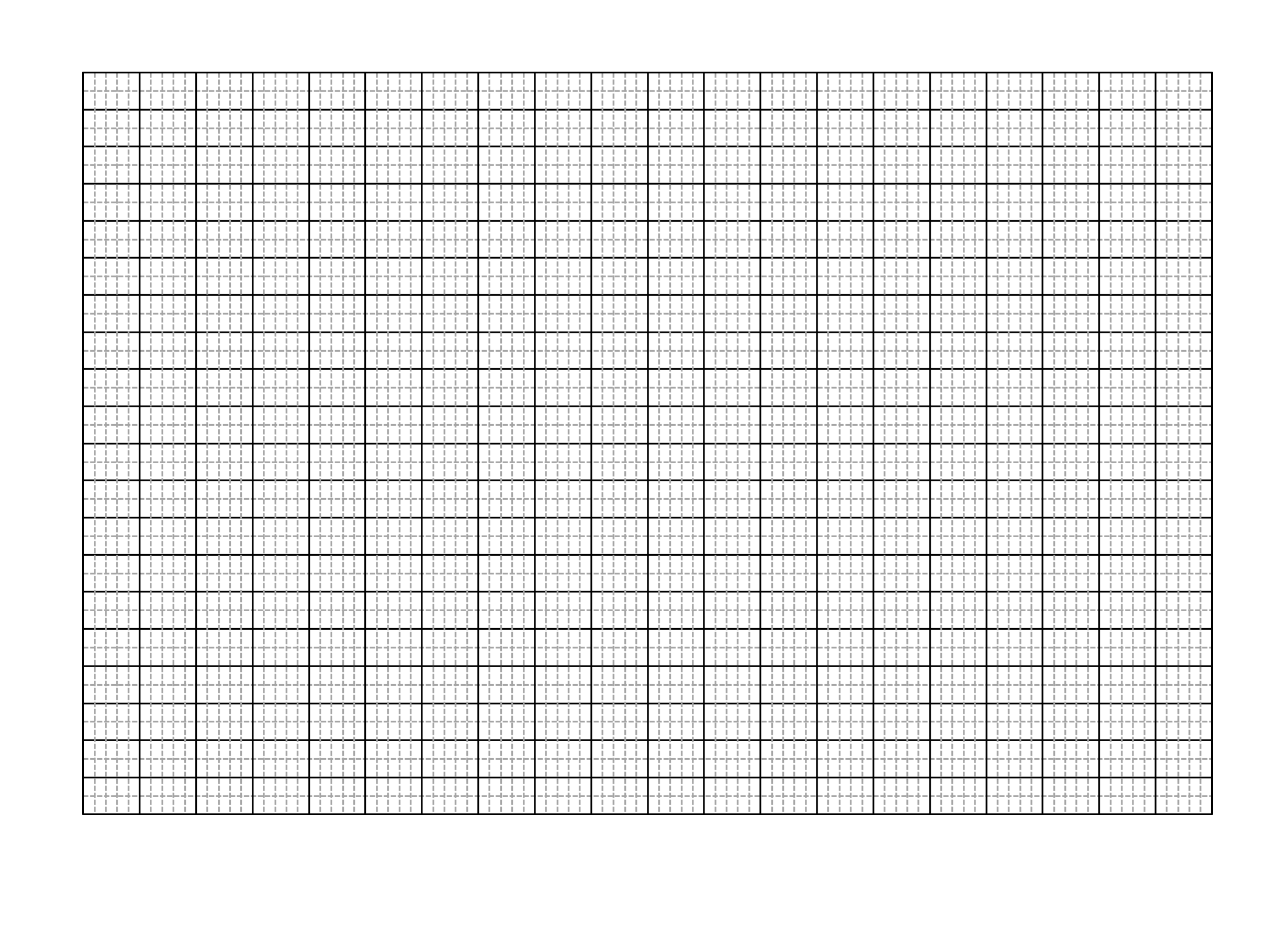6 (b) 80 mL of 0.05 M potassium hydroxide and 20 mL of 0.06 M calcium nitrate is mixed at 70°C. Predict if a precipitate of calcium hydroxide will be observed in the resultant mixture.Support your answer with relevant calculations. 2 (c) Acidic mine tailings, which consist of process effluents generated in mineral processing, can be treated with calcium hydroxide to reduce acidity and help with removal of heavy metals such as iron(III) ions from the wastewater.Explain the treatment of iron(III) contaminated tailings using calcium hydroxide with reference to solubility. Include relevant chemical equation. 2

### Question 26 (4 marks)

Formic acid (HCOOH) is an antibacterial agent that is also found in nature in most ants and stringless bees as an agent used on their prey or to defend the nest.

A 0.52 M solution of formic acid has a pH of 2.02. Calculate the pKb of the formate ion.

### Question 27 (8 marks)

Ethanol-blended fuel is widely used around the world as a biofuel additive for gasoline in vehicles.

 (a) Outline a method that can be used to produce ethanol. Support your answer with at least ONE chemical equation. 3 (b) Write a balanced chemical equation for combustion of ethanol. 1 (c) The following data applies to combustion of ethanol at 25°C:ΔcH° = − 1367 kJ mol-1ΔcS° = 182.49 J K-1 mol-1Calculate the change in Gibbs free energy for combustion of ethanol at 25°C and explain the spontaneity of the ethanol combustion. 4

### Question 28 (4 marks)

Calculate the pOH of the resulting solution after 35.0 mL of 0.01 M strontium hydroxide is added to 60.0 mL of 0.05 M nitric acid.

### Question 29 (10 marks)

In pyrometallurgical processing of copper(II) carbonate minerals, the ores are thermally decomposed in the roasting process to form copper(II) oxide. The copper(II) oxide can then be dissolved in sulfuric acid to give the corresponding copper(II) salt which can be refined further to give copper metal.

In laboratory, 25.5 g copper(II) oxide sample was leached in the sulfuric acid. The resulting solution was made up to 500 mL with distilled water. A colorimeter was used to measure the absorbance of the final solution to be 0.95.

The table shows the absorbance of various concentrations of copper sulfate standard solutions at  λmax​ of 635 nm.

 Concentration of CuSO4(aq) (mol L-1) Absorbance 0.00 0.00 0.10 0.25 0.20 0.60 0.30 0.85 0.40 1.10 0.50 1.40

 (a) Write a balanced chemical equation for sulfuric acid leaching of copper(II) oxide. 1 (b) On the grid, construct a calibration curve from the data given above and calculate the percentage of copper in the copper(II) oxide. 6 (c) Copper(II) oxide is an example of a basic oxide. Oxides of elements near the semi-metal region of the periodic table such as Al2O3 is an example of amphoteric oxide.Define the term amphoteric. Include at least TWO balanced chemical equations in your answer. 3

### Question 30 (4 marks)

Primary, secondary and tertiary alcohols of the same carbon length have quite different boiling points.

Explain the difference in boiling point of these organic compounds, showing all intermolecular forces. Support your answer with diagrams.

### Question 31 (6 marks)

The structure of the synthetic detergent is shown below.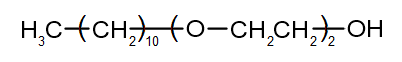(a) Identify the type of this detergent. 1 (b) Compare the structure of this detergent to the structure of soap. 2 (c) Account for the cleaning action of this detergent in terms of chemical properties. 3

### Question 32 (8 marks)

Compound X is a haloalkane of molar mass 169.986 g mol-1. It has the elemental composition of 21.2% carbon, 4.2% hydrogen and 74.6% iodine by mass.

The compound X underwent substitution reaction to produce compound Y. Treatment of compound Y with an oxidising agent slowly formed a single organic compound.

 (a) Outline the reagents and conditions required for each step, showing the structural formulae of the products formed. 5 (b) Name the compounds X, Y and Z. 3

## See detailed solutions to the 2020 HSC Chemistry Trial Exam Paper by creating your free account